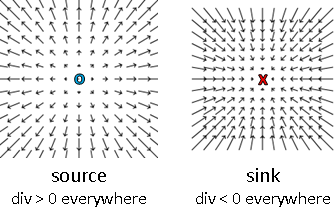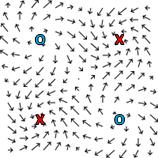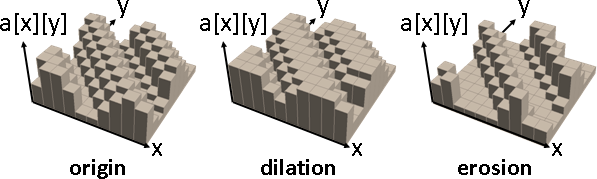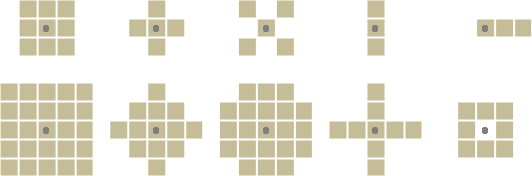Level Set (ℝⁿ ⇨ ℝ)（Under Construction!）

Level Set（Contour Line）（Isoline）

「等高線」。函數曲線一樣高的地方。習慣畫成俯瞰平面圖。f(x,y)是一群等高線。f(x,y) = 0是一個等高線。f(x,y) ≥ 0是一群等高線，形成區域，區分內部外部。https://en.wikipedia.org/wiki/Test_functions_for_optimization

Monotone Function：單調函數。遞增、遞減。

Convex Function：凸函數。斜率是單調函數，遞增是凸、遞減是凹。

Unimodal Function：單峰函數。先遞增（減）再遞減（增）。

Quasiconvex Function：擬凸函數。等高線是凸的，遞增是擬凸、遞減是擬凹。

https://mjo.osborne.economics.utoronto.ca/index.php/tutorial/index/1/qcc/t
f(a,x) = ax  在第一三象限是擬凹函數、第二四象限是擬凸函數
https://www.desmos.com/calculator/vkru83pare

[A,X] = meshgrid(-10:0.5:10,-10:10);
Z = A .* X;
surf(A,X,Z)

f(a,x) = (ax)^2 四個象限都是擬凹函數，合起來看像個碗（假的凸函數），截面是拋物線
https://www.desmos.com/calculator/roky6zr9lf

[A,X] = meshgrid(-10:0.5:10,-10:10);
Z = A .* X;
Z = Z .* Z;
surf(A,X,Z)

Level Set (ℝⁿ ⇨ ℝᵐ)（Under Construction!）

Level Set

Increasing Function ⮕ Positive Semidefinite Matrix

f(b) - f(a)
function f is monotone iff ――――――――――― ≥ 0
b - a

multivariate function f is monotone iff (b - a) ∙ (f(b) - f(a)) ≥ 0

Increasing Function ⮕ Nonnegative Divergence

function f is monotone iff d/dx f(x) ≥ 0

multivariate function f is monotone iff [ ∂/∂x ] ∙ [ f(x) ] ≥ 0
[ ∂/∂y ]   [ f(y) ]Lipschitz Function ⮕ Johnson–Lindenstrauss Lemma

(1-δ) ‖x-y‖² ≤ ‖f(x)-f(y)‖² ≤ (1+δ) ‖x-y‖²

JL矩陣：向量經過變換，長度平方差異在δ倍以內。

Lipschitz Function ⮕ Diagonally Dominant Matrix

monotone: 0 ≤ (f(b) - f(a)) / (b - a)
Lipschitz: -1 ≤ (f(b) - f(a)) / (b - a) ≤ +1
positive Lipschitz => monotone
|f(a) + f(b)| ≤ |a + b|   其中一個變數變號
diagonally dominant matrix = 每個橫條都是Lipschitz function
(對於橫條的非主對角線元素來說。)
(例如Gauss–Seidel，更新x，方程式5x + y = 5是陡峭的，很近就撞到，所以收斂。
(更新式x = 1 - 0.2y，對y而言，斜率-1 ≤ -0.2 ≤ 1是平緩的。)
positive diagonally dominant => positive definite => monotone

Convex Function ⮕ Submodular Function

《Learning Submodular Functions》
Submodularity is in many ways similar to concavity of functions
defined on Rn. For example, concavity of differentiable functions
is equivalent to gradient monotonicity, and submodularity is equivalent
to monotonicity of the marginal values:

• Non-negative if f(S) ≥ 0 for all S.
• Monotone (or non-decreasing) if f(S) ≤ f(T) for all S ⊆ T.
• Submodular if f(A) + f(B) ≥ f(A ∪ B) + f(A ∩ B) ∀A,B ⊆ [n].
or equivalently f(A ∪ {i}) − f(A) ≥ f(B ∪ {i}) − f(B)
for all A ⊆ B ⊆ [n] and i ∉ B.
• Subadditive if f(S) + f(T) ≥ f(S ∪ T) for all S,T ⊆ [n].
• L-Lipschitz if |f(S + x) − f(S)| ≤ L for all S ⊆ [n] and x ∈ [n].

Isocline (ℝⁿ ⇨ ℝⁿ)（Under Construction!）

IsoclineIsocline

time series graph   函數曲線。以時間為主軸。
phase portrait      移動路線。初始條件(x₀,y₀)沿著向量場跑。
Nodal Set：特徵函數，高度為零的等高線。
Nullcline：梯度場，向量為零的等高線。
Streamline：梯度場的向量的切線方向連線；勢能場的等高線的垂直方向。
isocline：導數相等之處。
nullcline：導數為零之處。
stable：停在穩態、不斷循環。
unstable：走向無限。

Mathematical Morphology

Mathematical MorphologyHistogram3D[{{0,0},{0,0},{0,0}},{1},Boxed->False,Axes->None]

【註：我製圖技術差，只好介紹陣列版本，而非函數版本。】

dilation：鄰近格子取最大值。功效是補厚。
erosion：鄰近格子取最小值。功效是削薄。
closing：先 dilation 再 erosion。
opening：先 erosion 再 dilation。
top-hat transform：原函數減掉 opening。
bottom-hat transform：closing 減掉原函數。UVa 12702